# Andhra Pradesh SSC Board Question Paper for Class 10th Maths Paper 2 2016 In PDF

## AP SSC or 10th Class Question Paper Mathematics Paper 2 English Medium 2016 with Solutions – Free Download

Andhra Pradesh SSC (Class 10) Maths 2016 question paper 2 with solutions are given here in a downloadable pdf format and also in the text so that the students can easily practise them. Along with the solutions, they can also get the Maths question paper 2 2016 class 10 SSC for reference. Students are able to access all the Andhra Pradesh board previous year Maths question papers here. AP 10th Class Mathematics Question Paper 2016 Paper 2 can be downloaded easily and students can use them and verify the answers provided by BYJU’S. Solving 2016 Maths question paper 2 for Class 10 will help the students to predict the type of questions and pattern that will appear in the exam.

### QUESTION PAPER CODE 16E(A)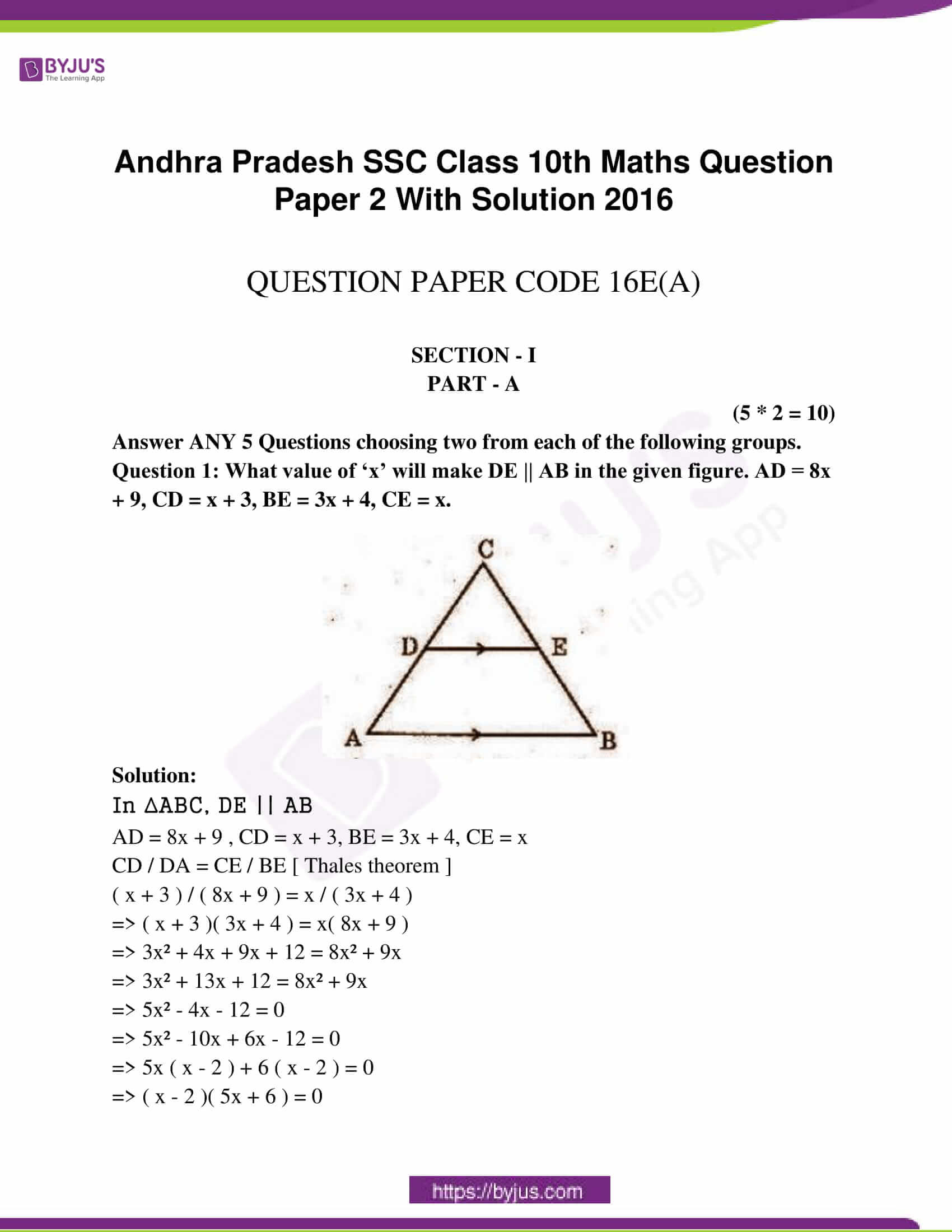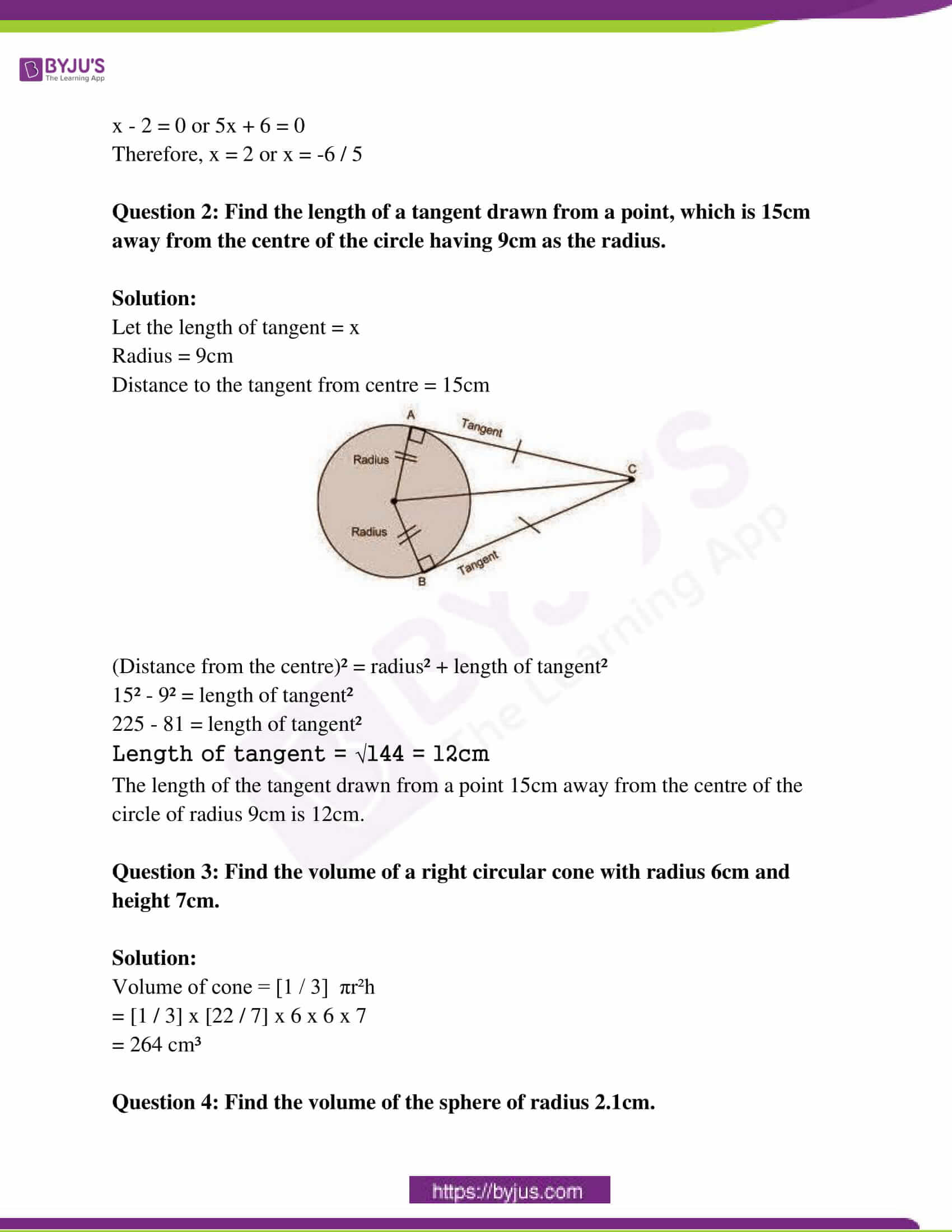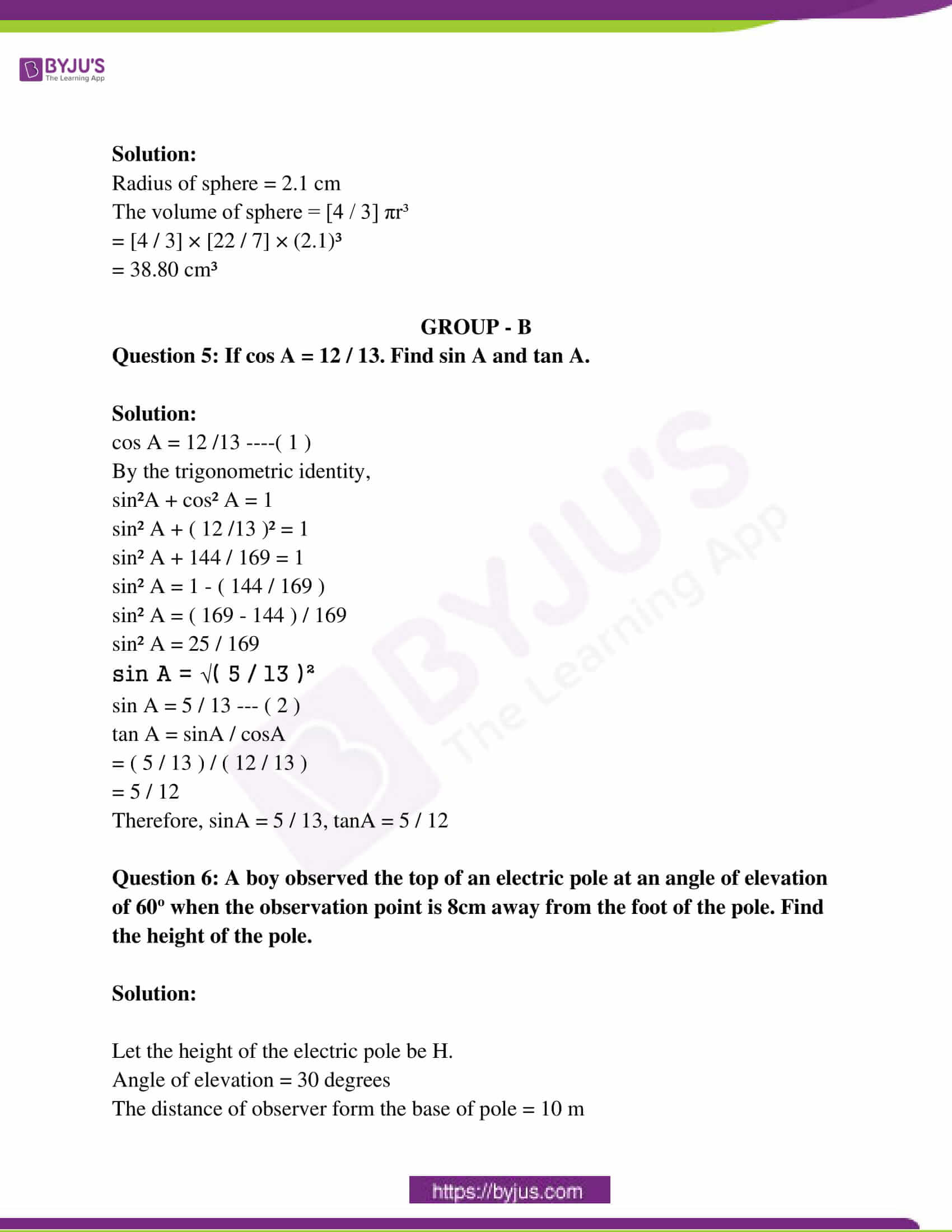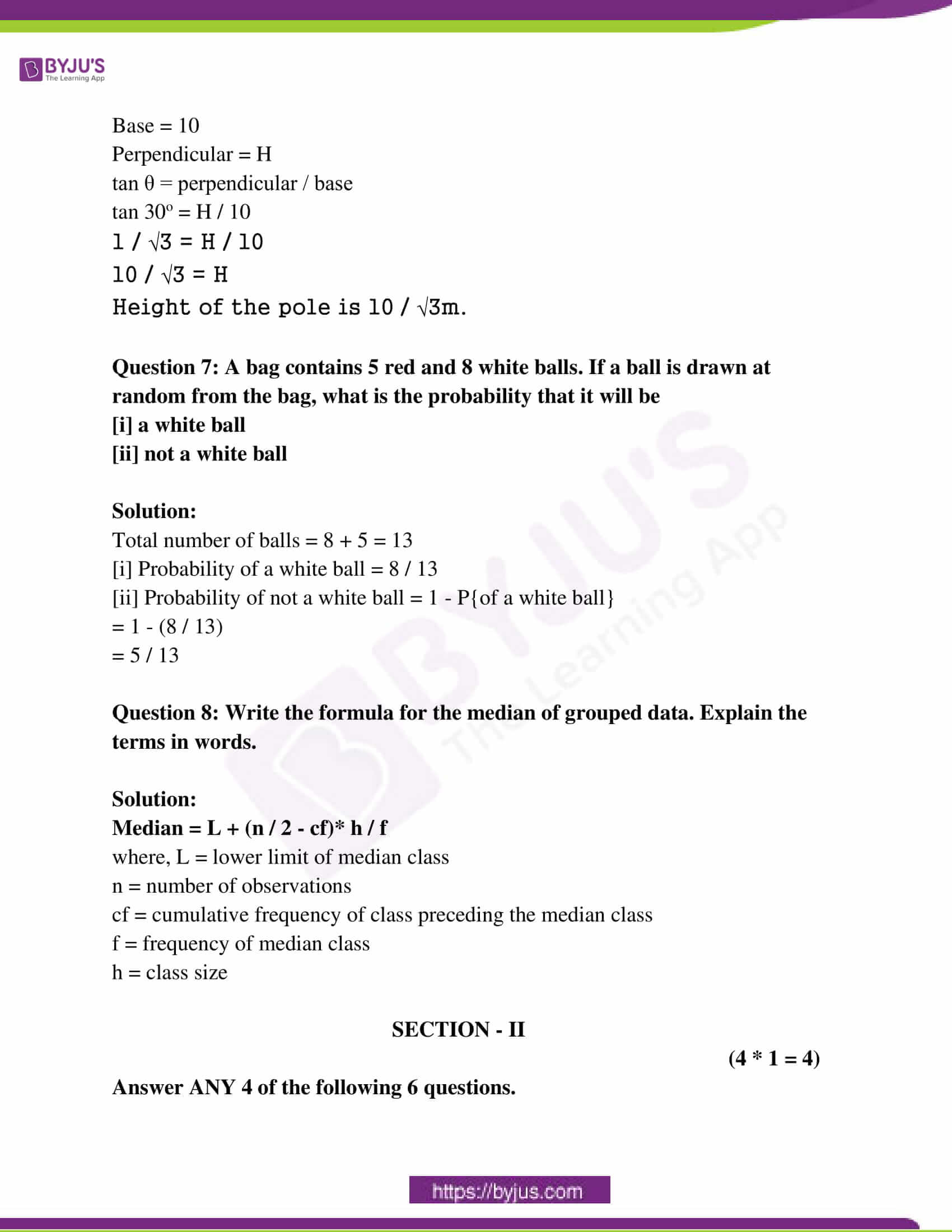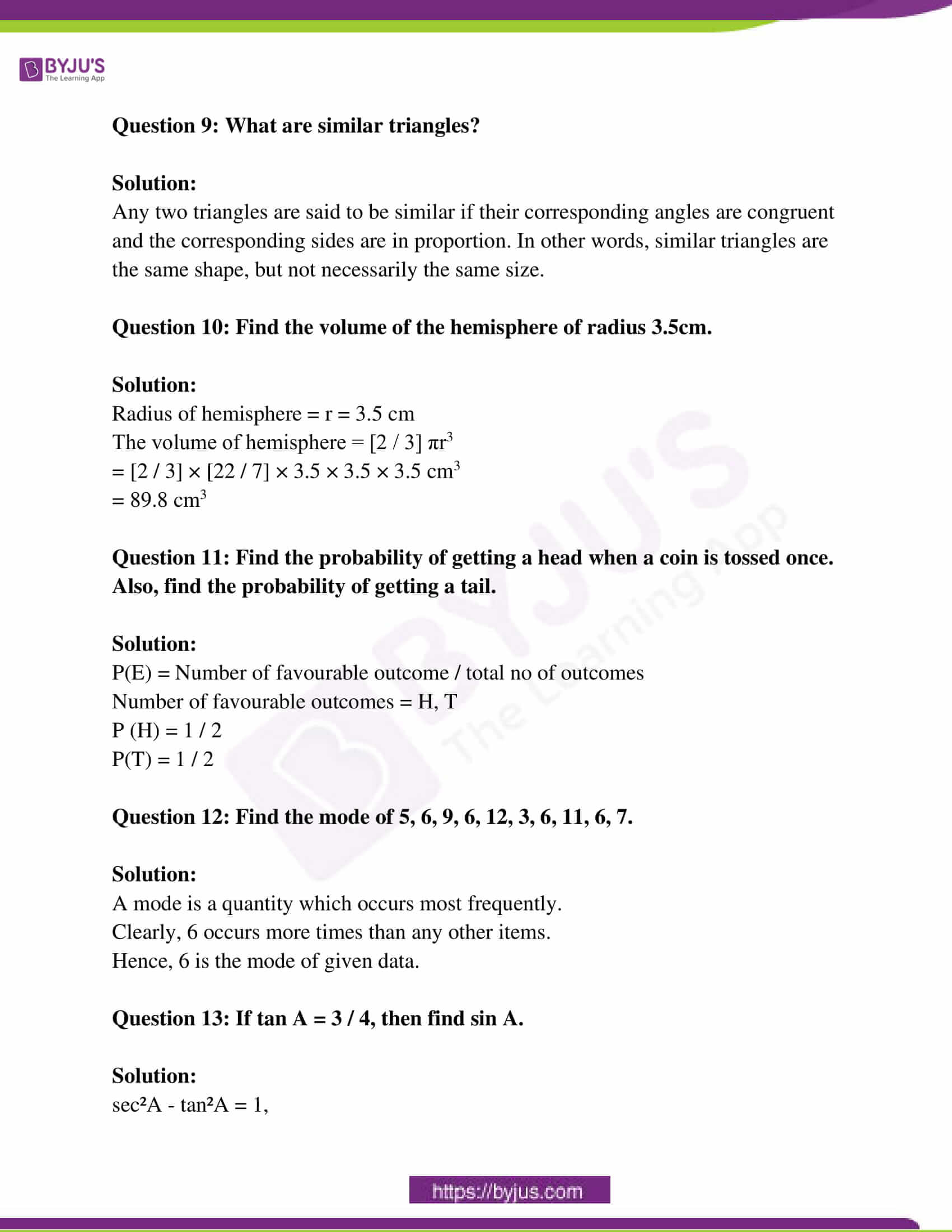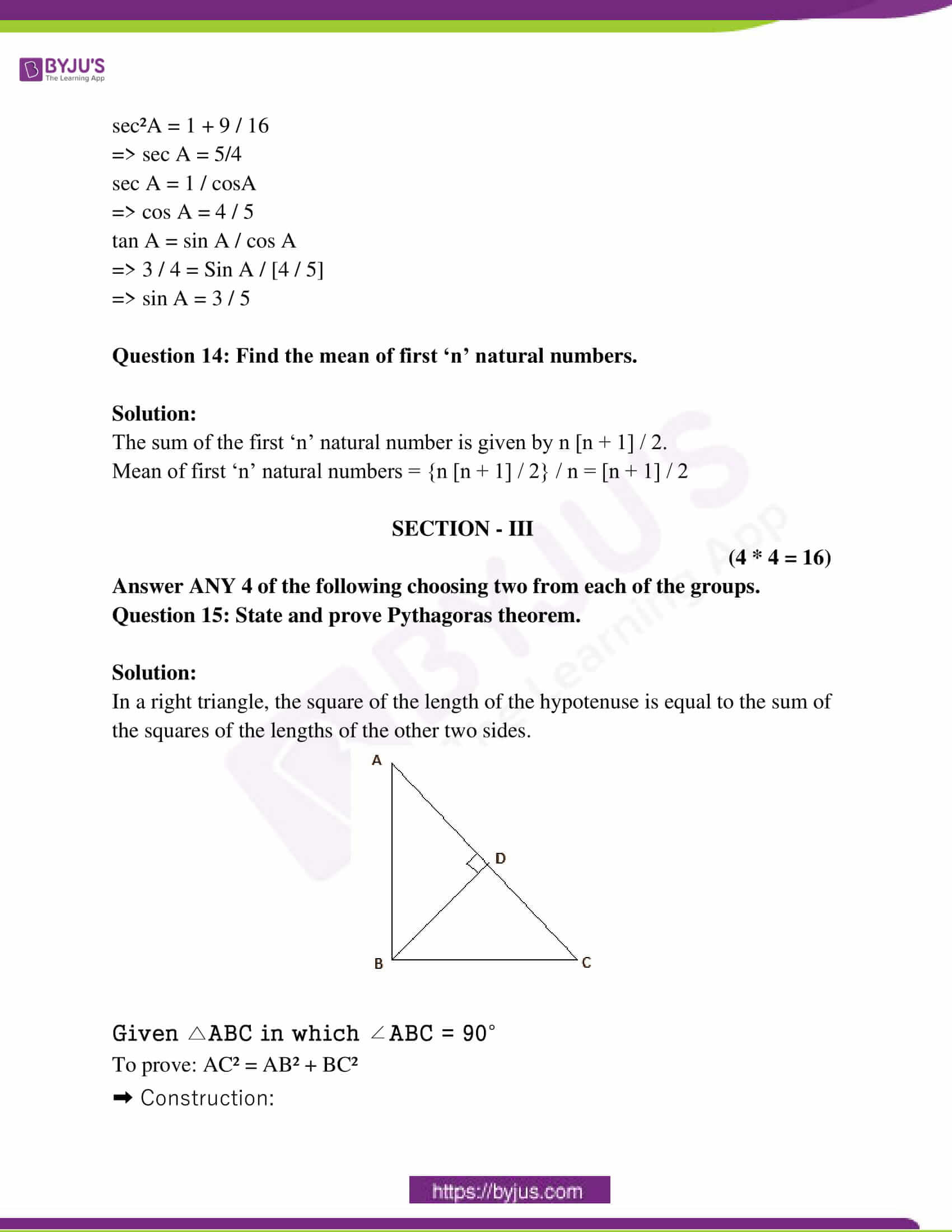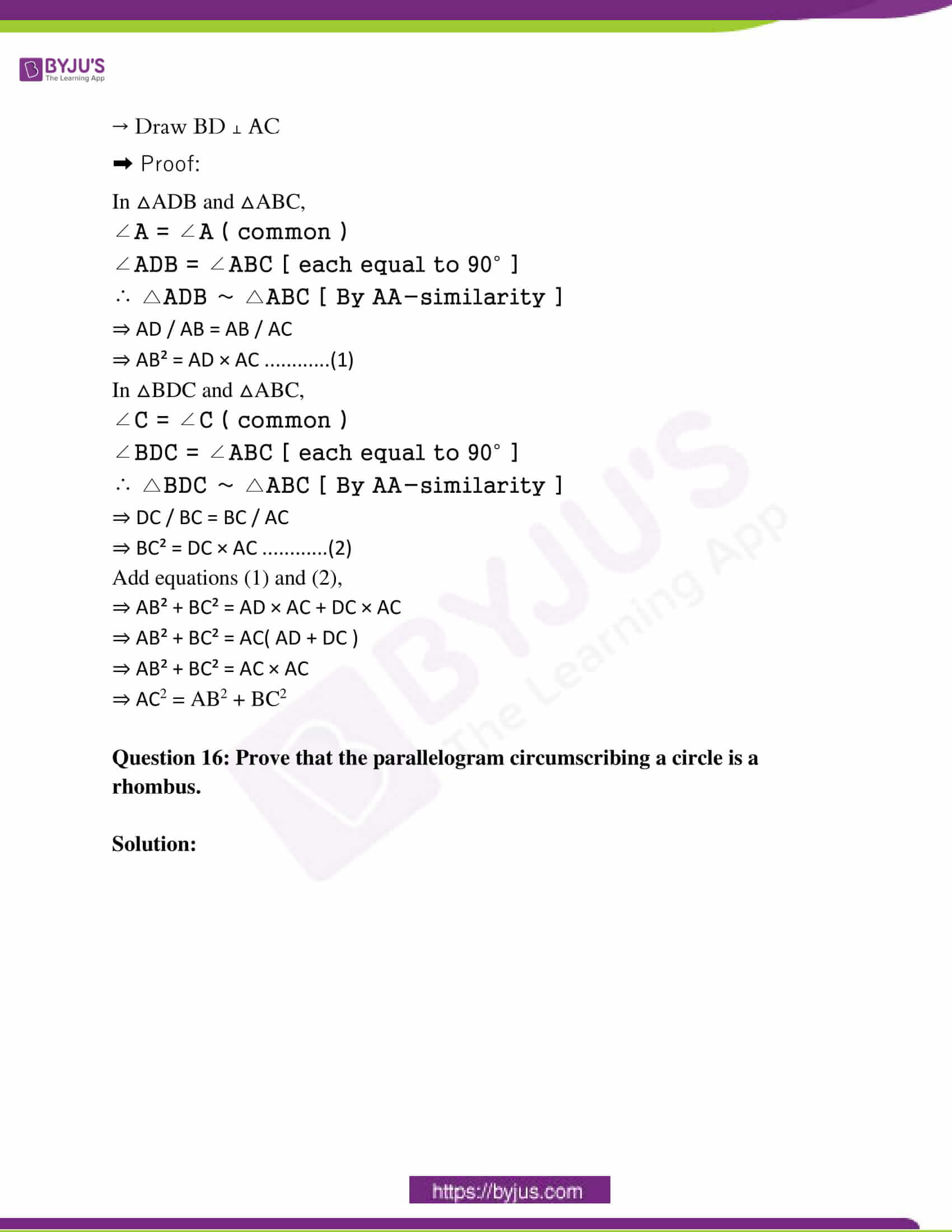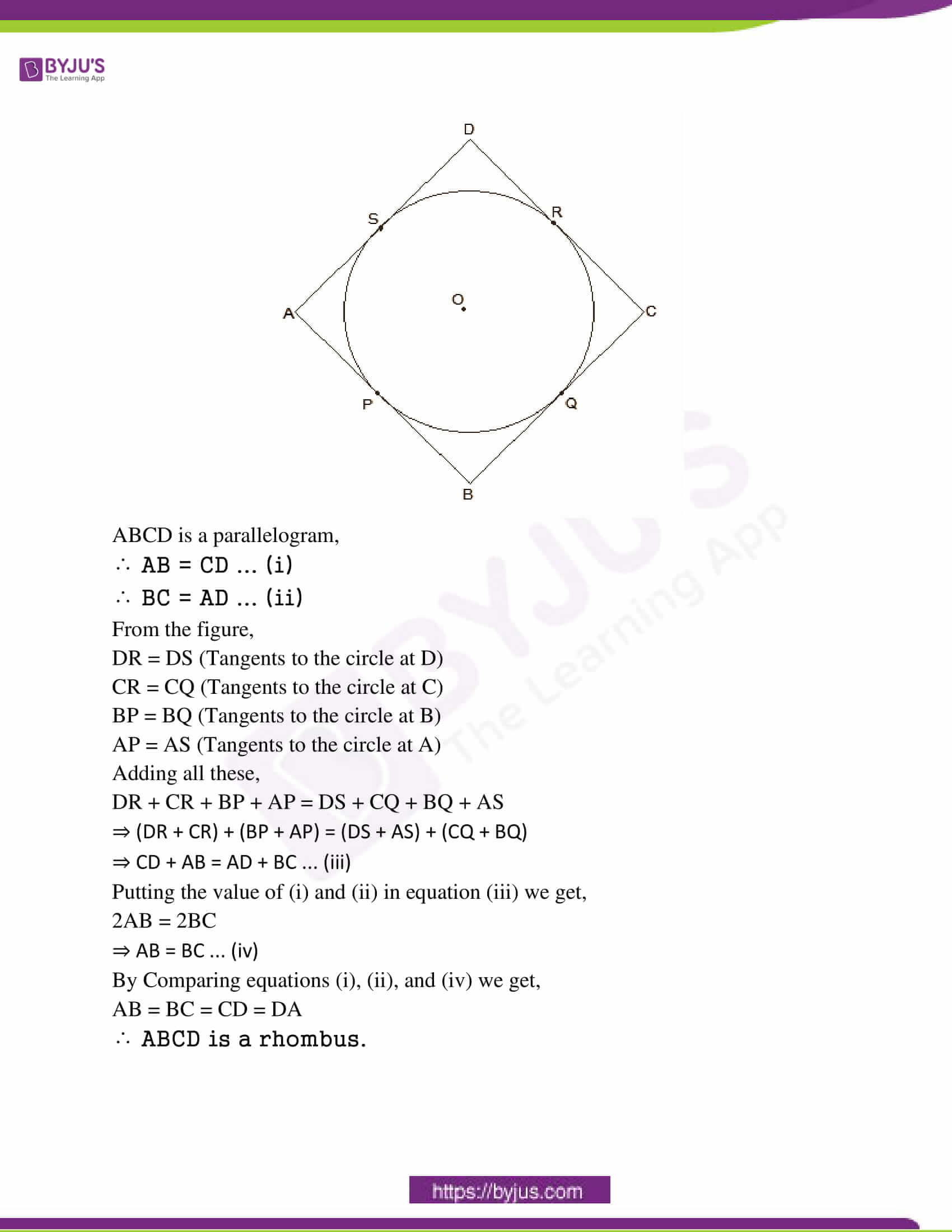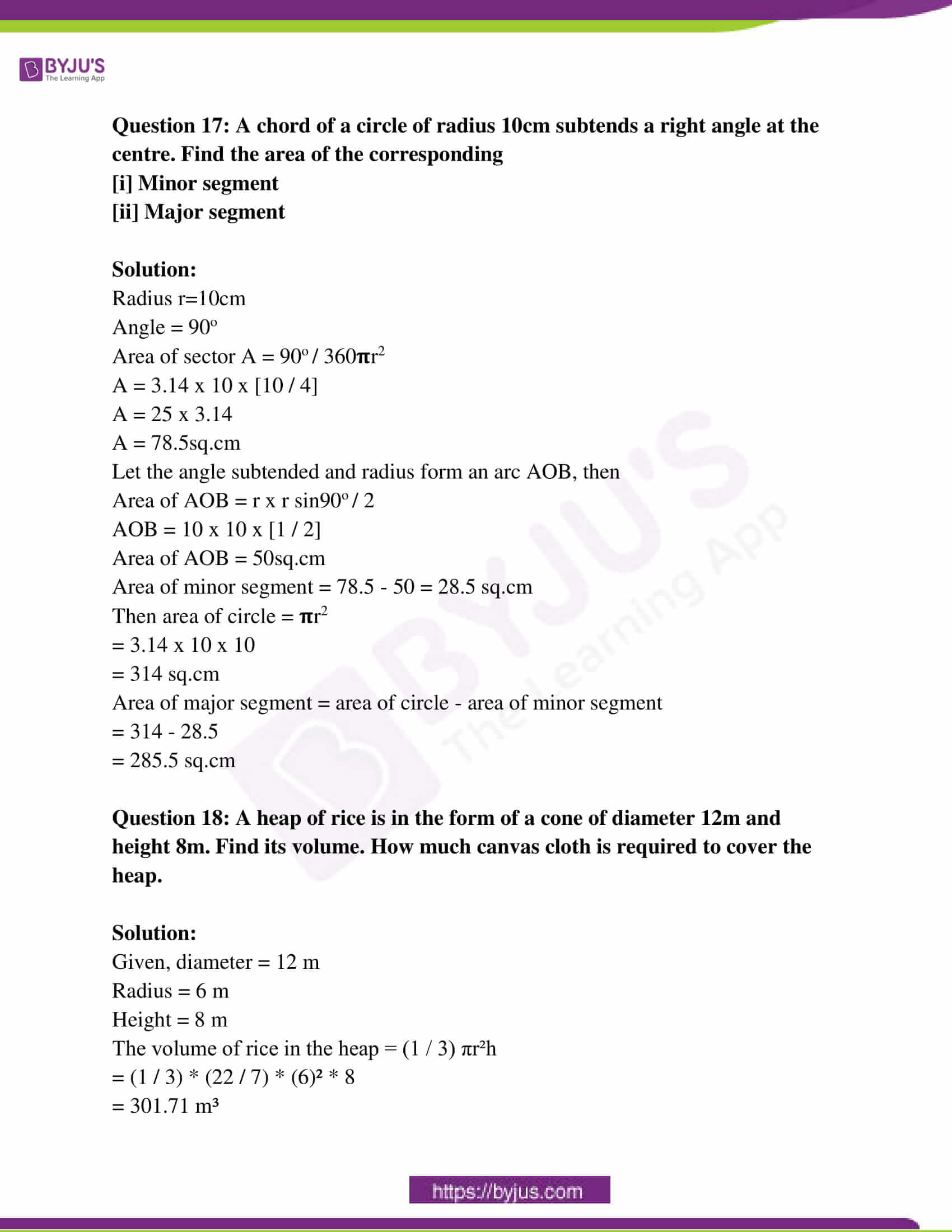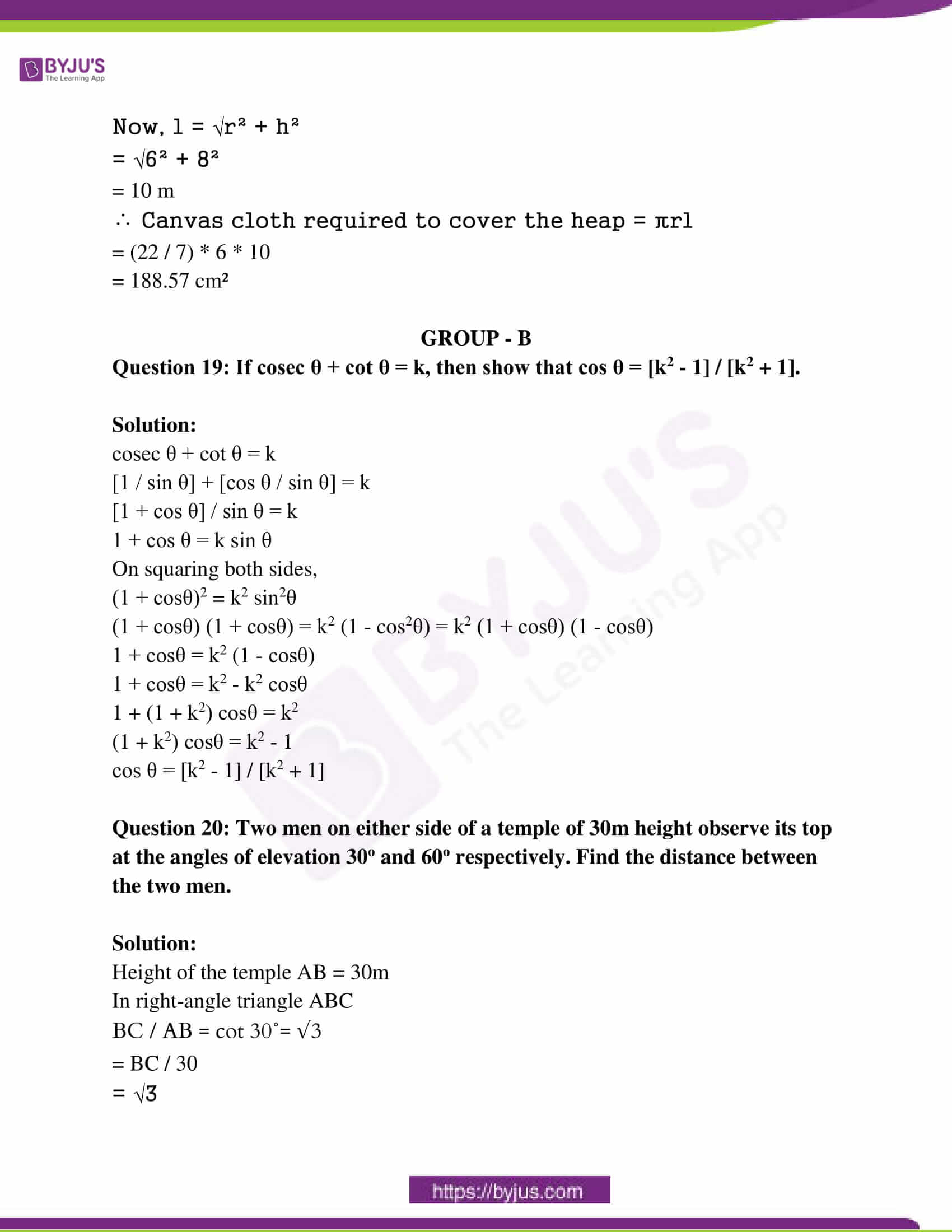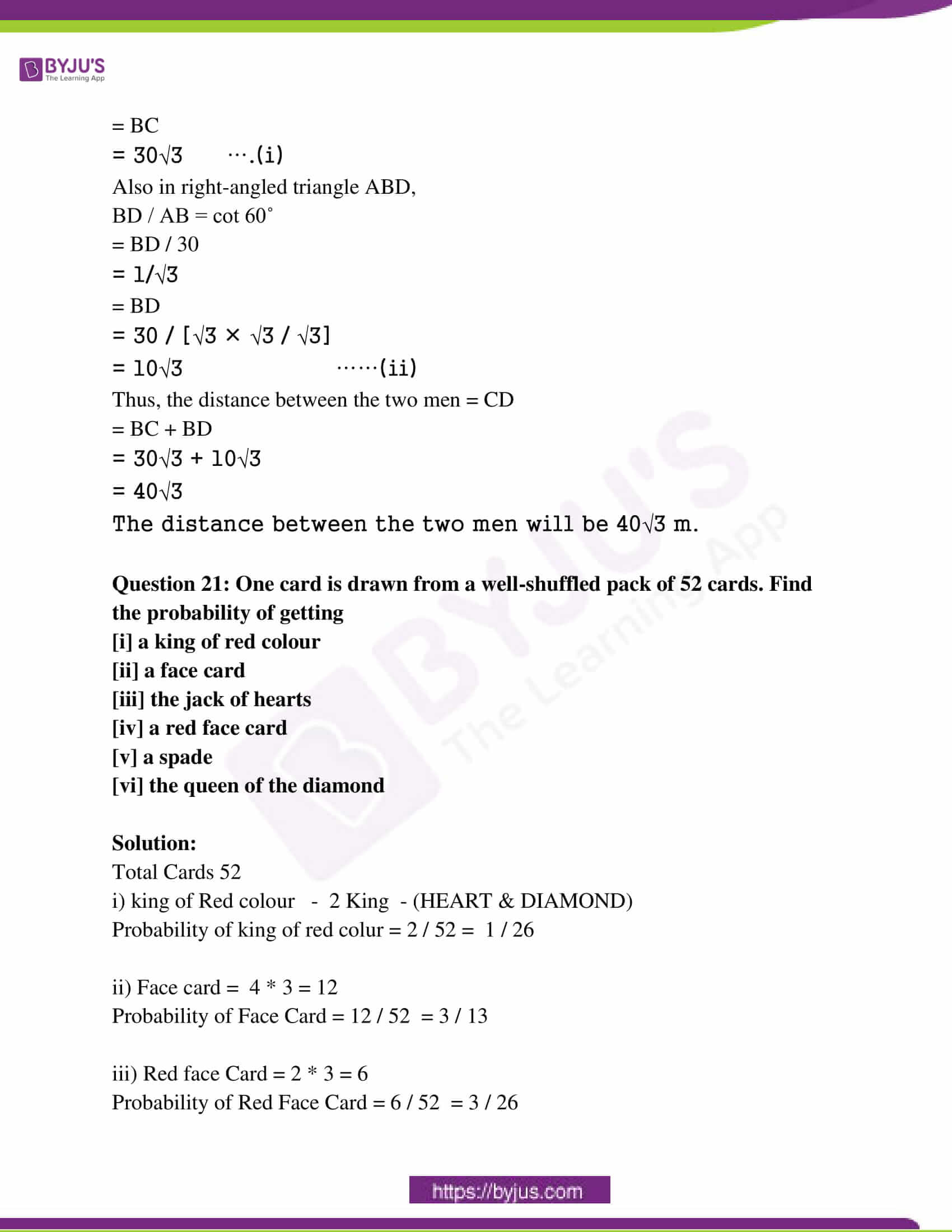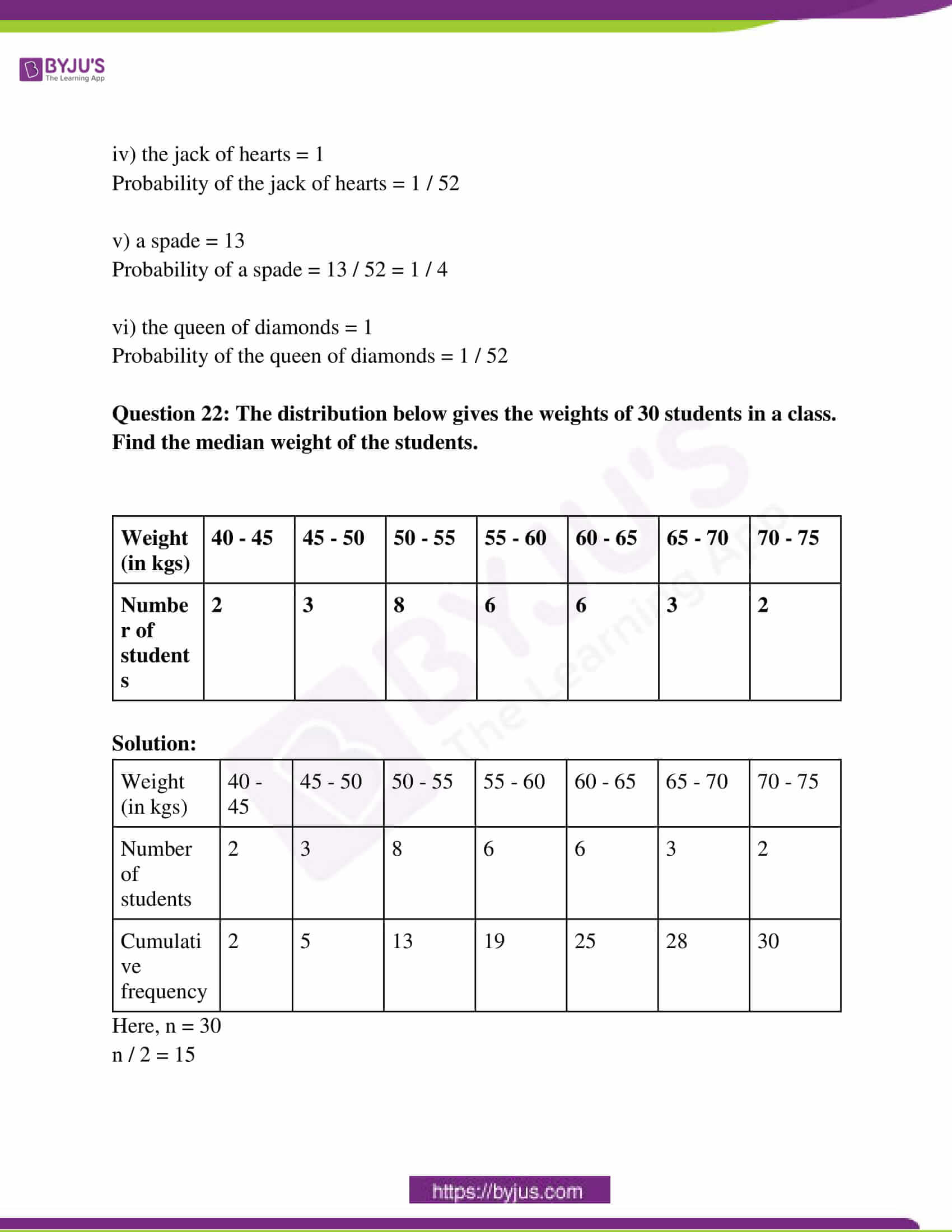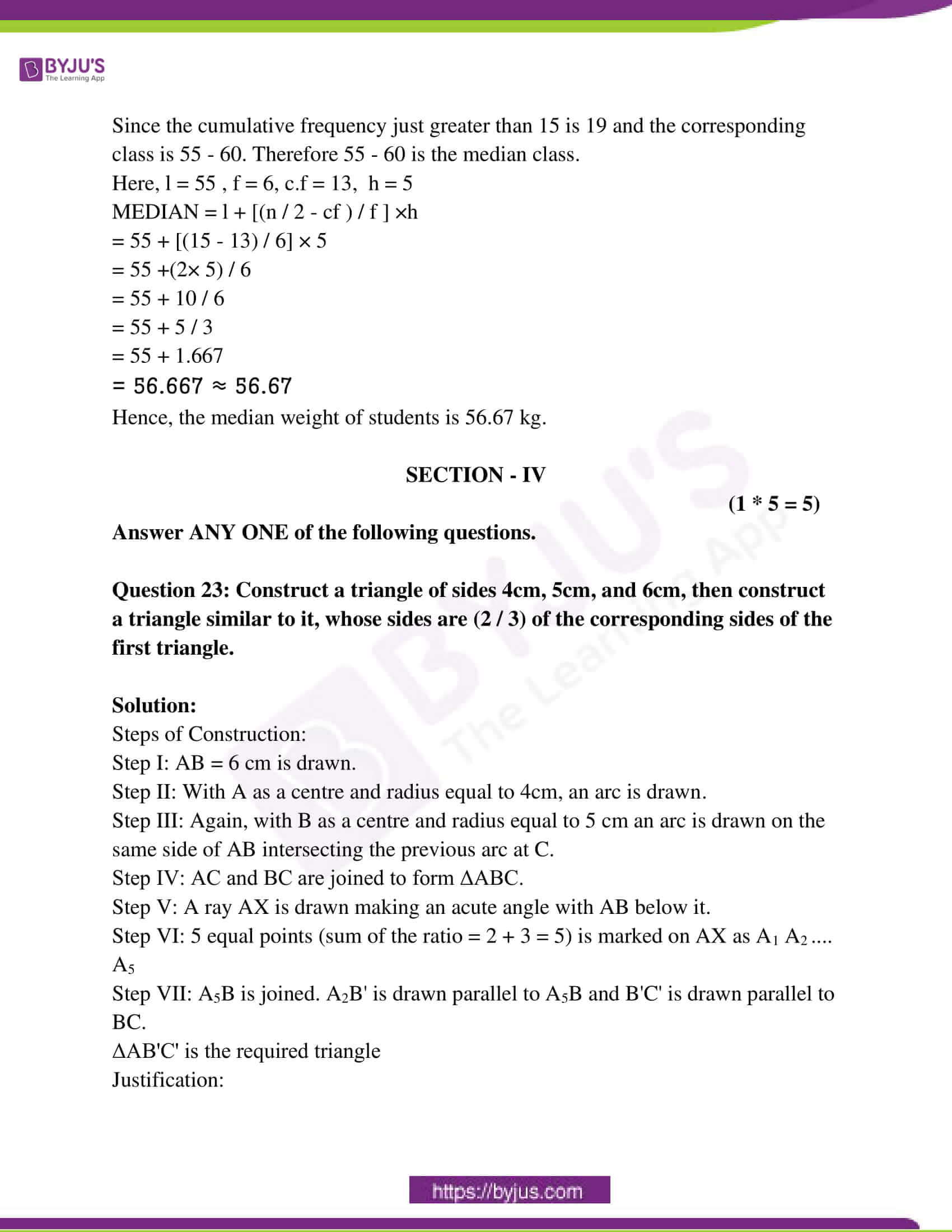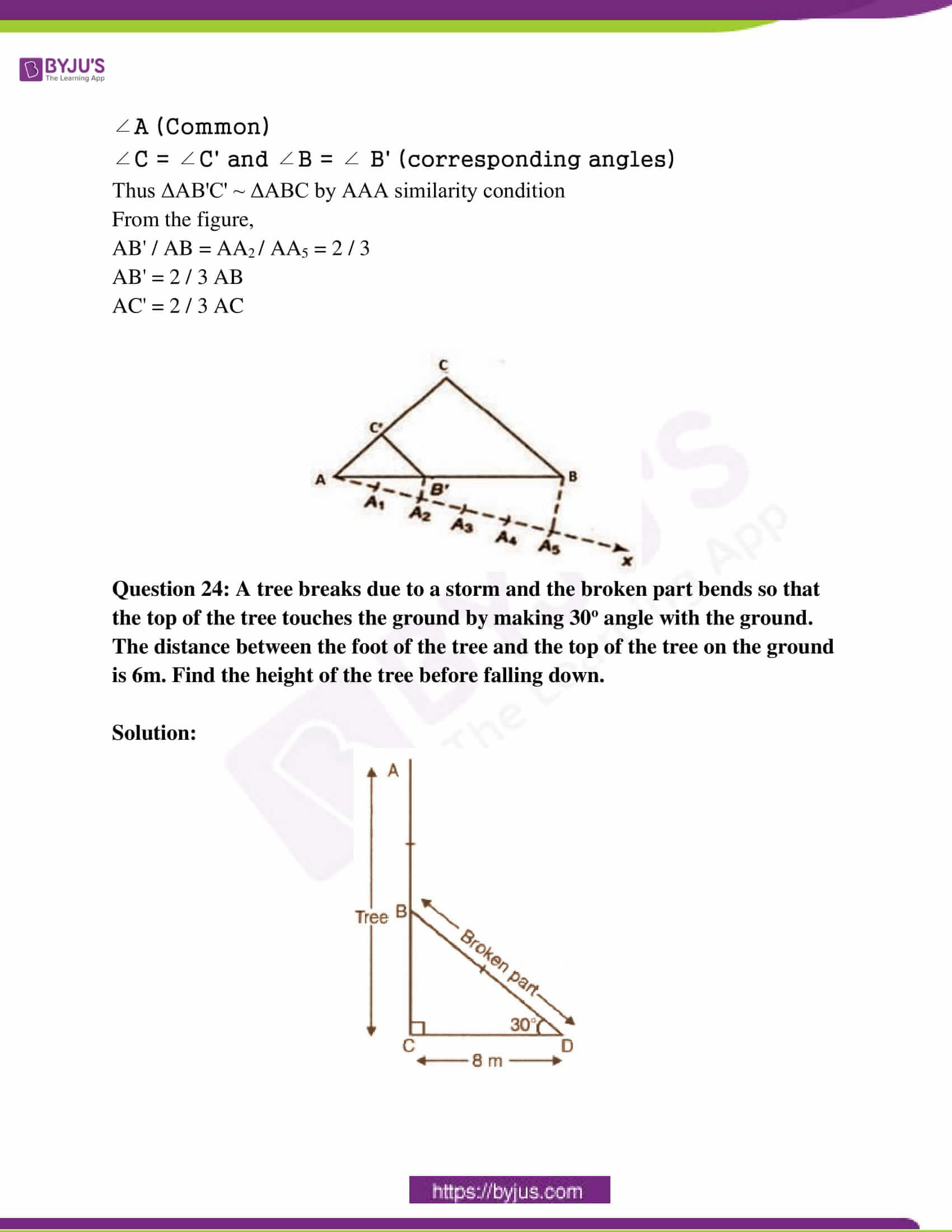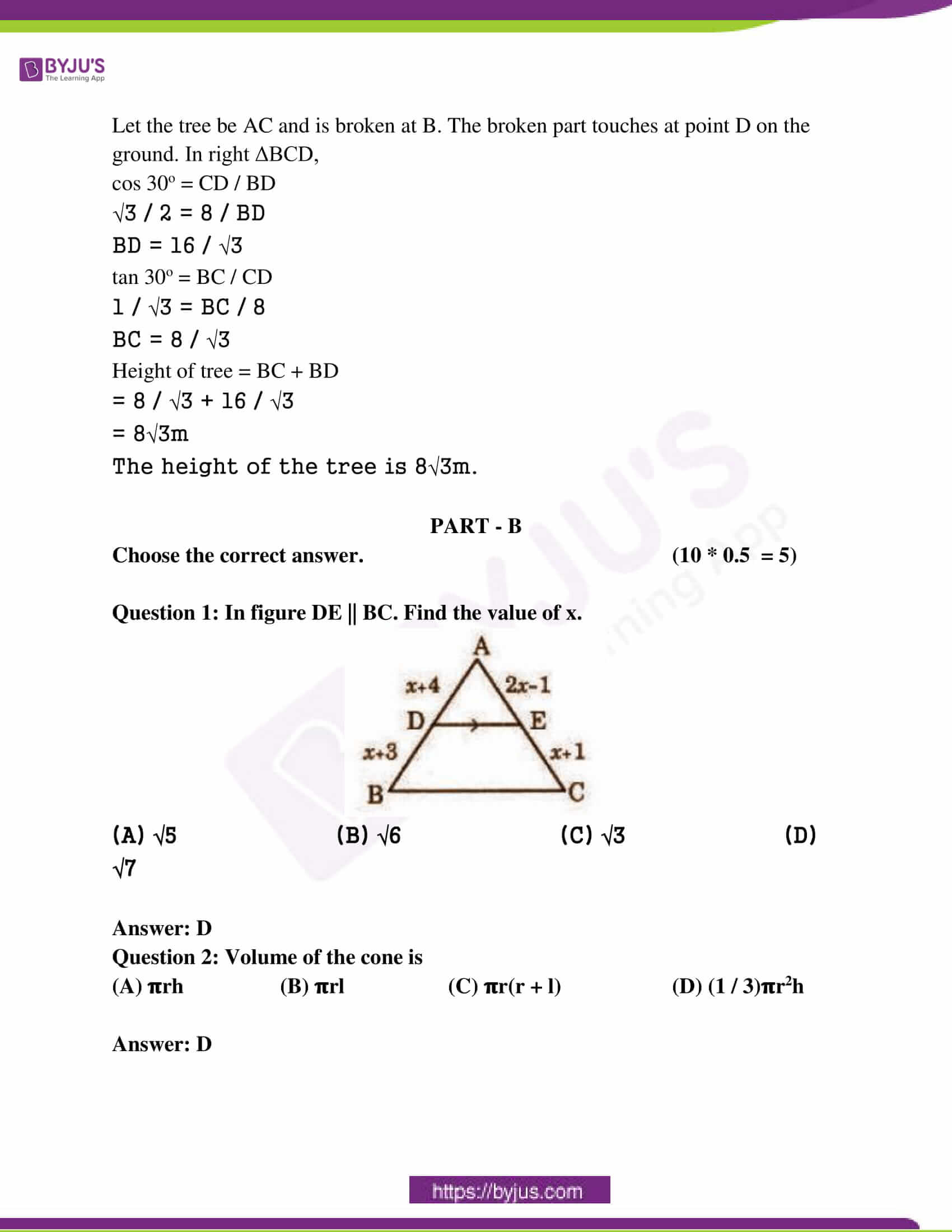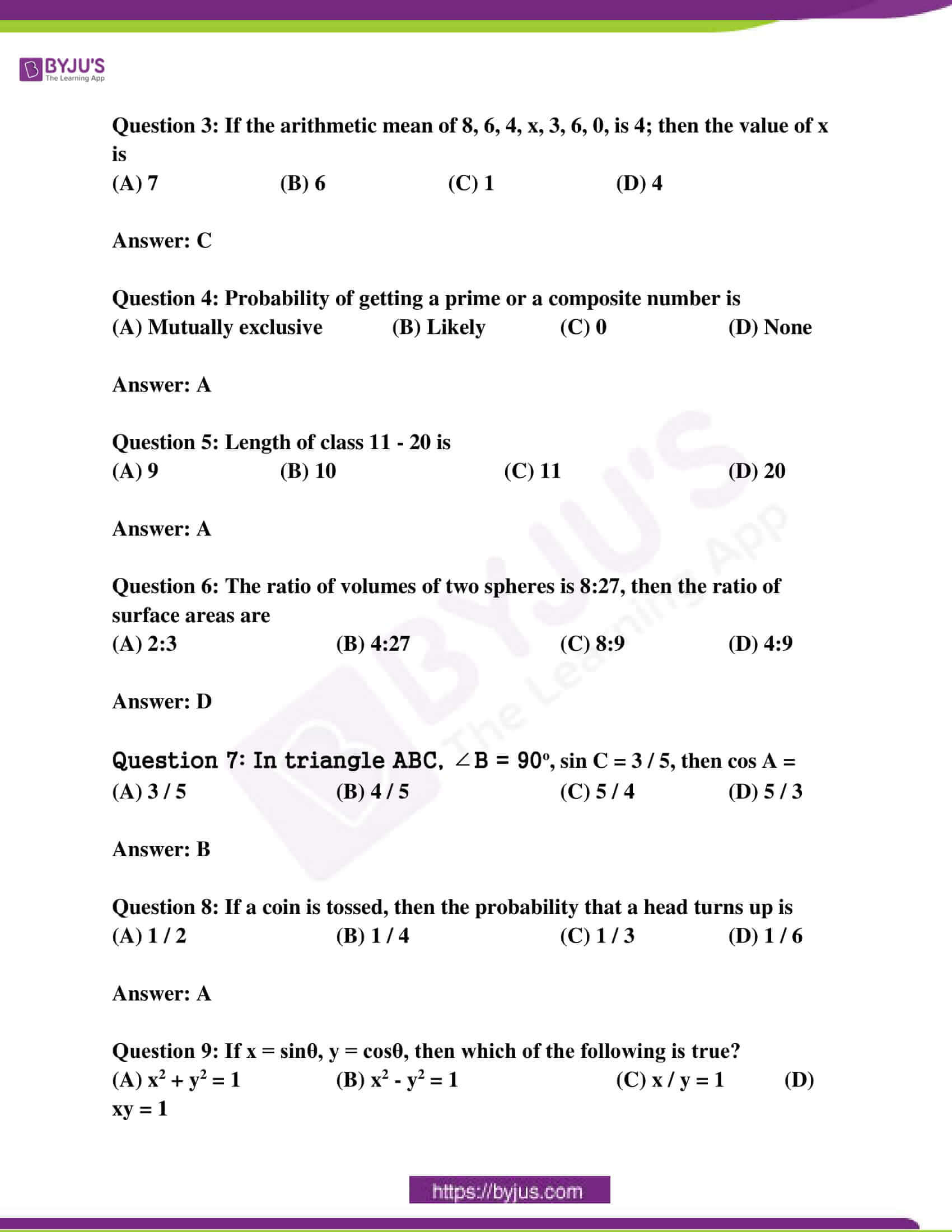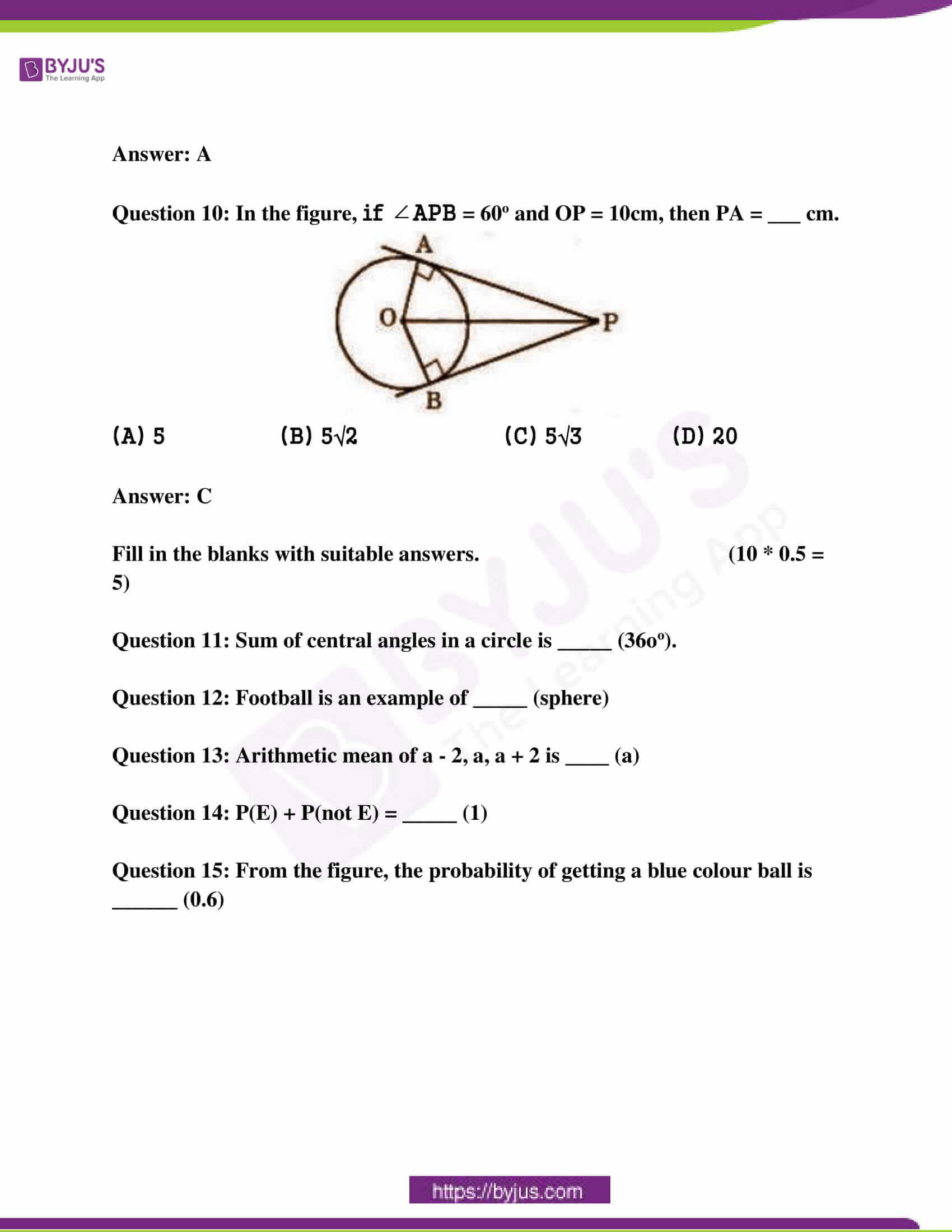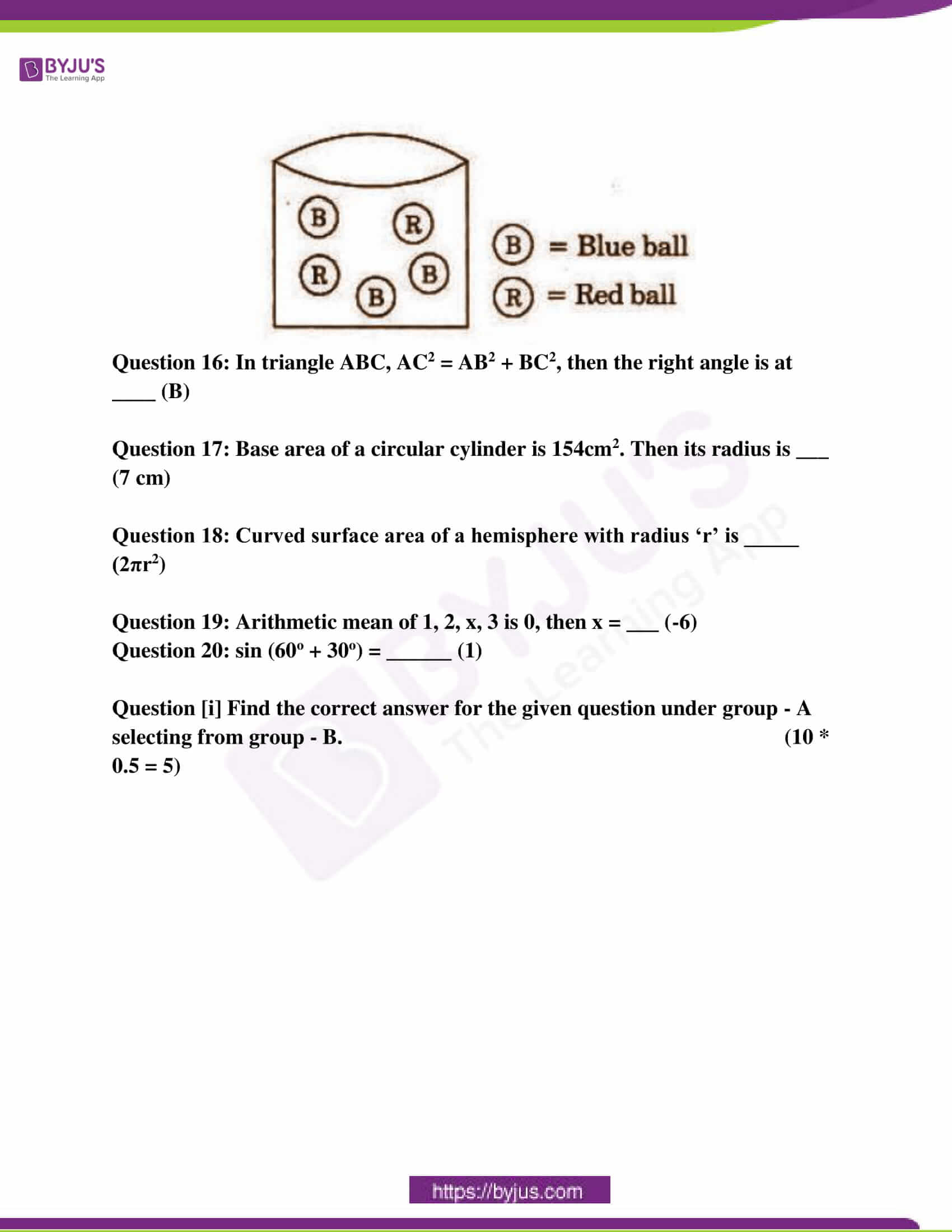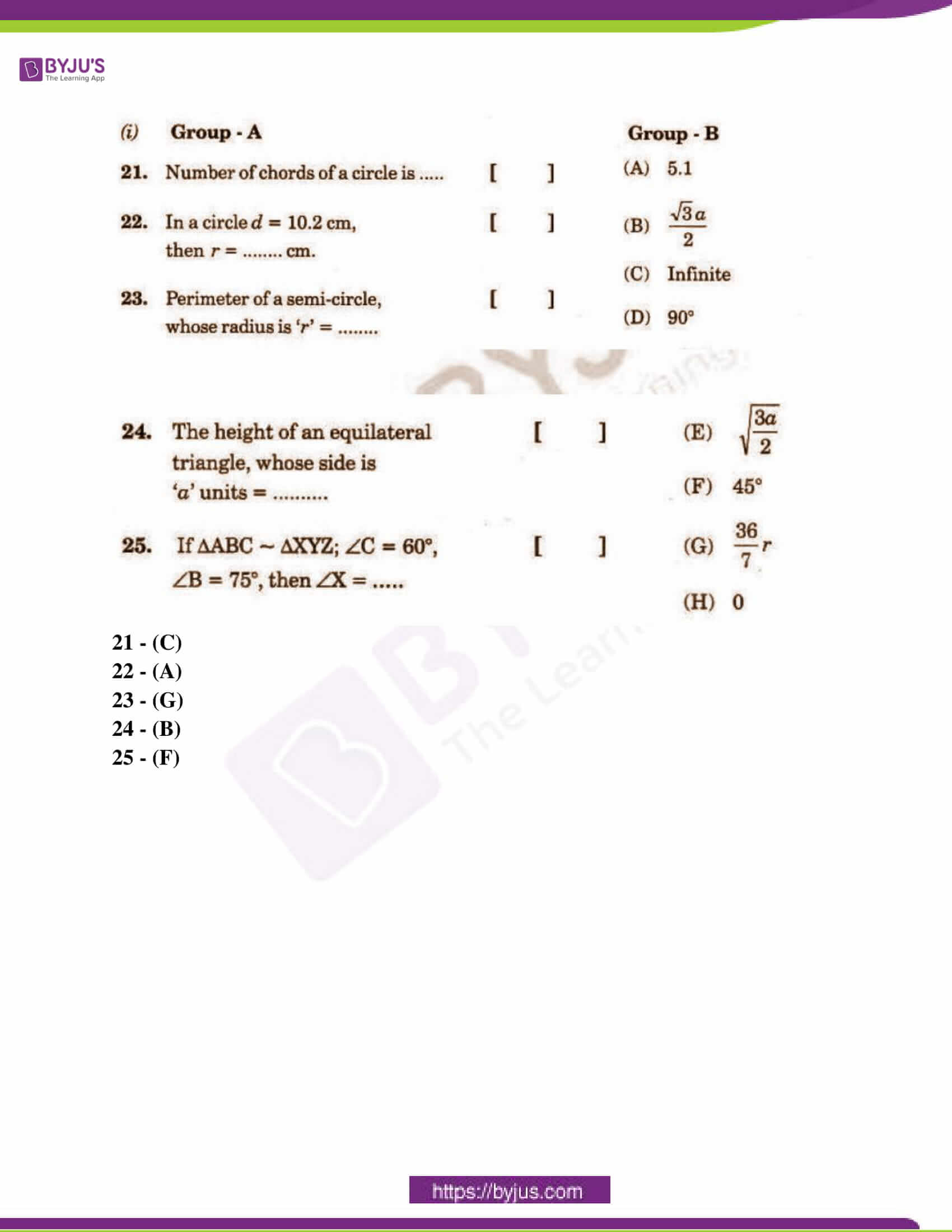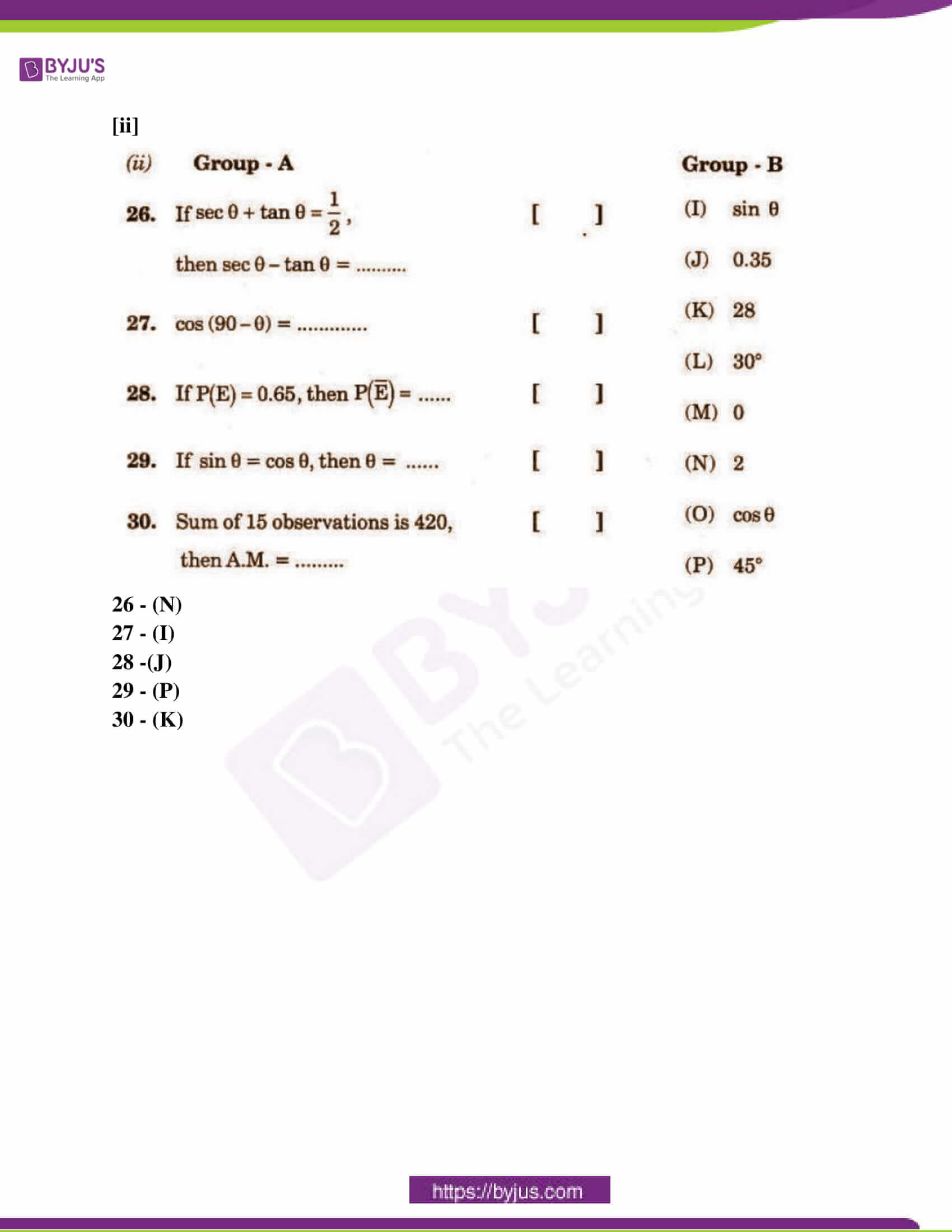SECTION – I

PART – A

(5 * 2 = 10)

Answer ANY 5 Questions choosing two from each of the following groups.

Question 1: What value of ‘x’ will make DE || AB in the given figure. AD = 8x + 9, CD = x + 3, BE = 3x + 4, CE = x?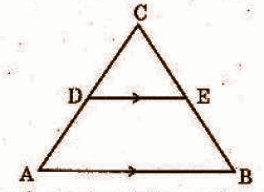Solution:

In ∆ABC, DE || AB

AD = 8x + 9 , CD = x + 3, BE = 3x + 4, CE = x

CD / DA = CE / BE [ Thales theorem ]

( x + 3 ) / ( 8x + 9 ) = x / ( 3x + 4 )

=> ( x + 3 )( 3x + 4 ) = x( 8x + 9 )

=> 3x² + 4x + 9x + 12 = 8x² + 9x

=> 3x² + 13x + 12 = 8x² + 9x

=> 5x² – 4x – 12 = 0

=> 5x² – 10x + 6x – 12 = 0

=> 5x ( x – 2 ) + 6 ( x – 2 ) = 0

=> ( x – 2 )( 5x + 6 ) = 0

x – 2 = 0 or 5x + 6 = 0

Therefore, x = 2 or x = -6 / 5

Question 2: Find the length of a tangent drawn from a point, which is 15cm away from the centre of the circle having 9cm as the radius.

Solution:

Let the length of tangent = x

Distance to the tangent from centre = 15cm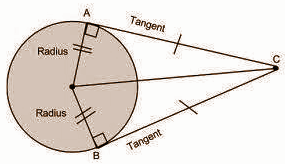(Distance from the centre)² = radius² + length of tangent²

15² – 9² = length of tangent²

225 – 81 = length of tangent²

Length of tangent = √144 = 12cm

The length of the tangent drawn from a point 15cm away from the centre of the circle of radius 9cm is 12cm.

Question 3: Find the volume of a right circular cone with radius 6cm and height 7cm.

Solution:

Volume of cone = [1 / 3] πr²h

= [1 / 3] x [22 / 7] x 6 x 6 x 7

= 264 cm³

Question 4: Find the volume of the sphere of radius 2.1cm.

Solution:

Radius of sphere = 2.1 cm

The volume of sphere = [4 / 3] πr³

= [4 / 3] × [22 / 7] × (2.1)³

= 38.80 cm³

GROUP – B

Question 5: If cos A = 12 / 13. Find sin A and tan A.

Solution:

cos A = 12 /13 —-( 1 )

By the trigonometric identity,

sin²A + cos² A = 1

sin² A + ( 12 /13 )² = 1

sin² A + 144 / 169 = 1

sin² A = 1 – ( 144 / 169 )

sin² A = ( 169 – 144 ) / 169

sin² A = 25 / 169

sin A = √( 5 / 13 )²

sin A = 5 / 13 — ( 2 )

tan A = sinA / cosA

= ( 5 / 13 ) / ( 12 / 13 )

= 5 / 12

Therefore, sinA = 5 / 13, tanA = 5 / 12

Question 6: A boy observed the top of an electric pole at an angle of elevation of 60o when the observation point is 8cm away from the foot of the pole. Find the height of the pole.

Solution:

Let the height of the electric pole be H.

Angle of elevation = 30 degrees

The distance of observer form the base of pole = 10 m

Base = 10

Perpendicular = H

tan θ = perpendicular / base

tan 30o = H / 10

1 / √3 = H / 10

10 / √3 = H

Height of the pole is 10 / √3m.

Question 7: A bag contains 5 red and 8 white balls. If a ball is drawn at random from the bag, what is the probability that it will be

[i] a white ball

[ii] not a white ball

Solution:

Total number of balls = 8 + 5 = 13

[i] Probability of a white ball = 8 / 13

[ii] Probability of not a white ball = 1 – P{of a white ball}

= 1 – (8 / 13)

= 5 / 13

Question 8: Write the formula for the median of grouped data. Explain the terms in words.

Solution:

Median = L + (n / 2 – cf)* h / f

where, L = lower limit of median class

n = number of observations

cf = cumulative frequency of class preceding the median class

f = frequency of median class

h = class size

SECTION – II

(4 * 1 = 4)

Answer ANY 4 of the following 6 questions.

Question 9: What are similar triangles?

Solution:

Any two triangles are said to be similar if their corresponding angles are congruent and the corresponding sides are in proportion. In other words, similar triangles are the same shape, but not necessarily the same size.

Question 10: Find the volume of the hemisphere of radius 3.5cm.

Solution:

Radius of hemisphere = r = 3.5 cm

The volume of hemisphere = [2 / 3] πr3

= [2 / 3] × [22 / 7] × 3.5 × 3.5 × 3.5 cm3

= 89.8 cm3

Question 11: Find the probability of getting a head when a coin is tossed once. Also, find the probability of getting a tail.

Solution:

P(E) = Number of favourable outcome / total no of outcomes

Number of favourable outcomes = H, T

P (H) = 1 / 2

P(T) = 1 / 2

Question 12: Find the mode of 5, 6, 9, 6, 12, 3, 6, 11, 6, 7.

Solution:

A mode is a quantity which occurs most frequently.

Clearly, 6 occurs more times than any other items.

Hence, 6 is the mode of given data.

Question 13: If tan A = 3 / 4, then find sin A.

Solution:

sec²A – tan²A = 1,

sec²A = 1 + 9 / 16

=> sec A = 5/4

sec A = 1 / cosA

=> cos A = 4 / 5

tan A = sin A / cos A

=> 3 / 4 = Sin A / [4 / 5]

=> sin A = 3 / 5

Question 14: Find the mean of first ‘n’ natural numbers.

Solution:

The sum of the first ‘n’ natural number is given by n [n + 1] / 2.

Mean of first ‘n’ natural numbers = {n [n + 1] / 2} / n = [n + 1] / 2

SECTION – III

(4 * 4 = 16)

Answer ANY 4 of the following choosing two from each of the groups.

Question 15: State and prove Pythagoras theorem.

Solution:

In a right triangle, the square of the length of the hypotenuse is equal to the sum of the squares of the lengths of the other two sides.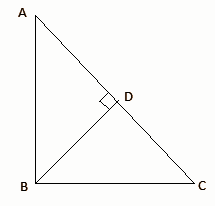Given △ABC in which ∠ABC = 90°

To prove: AC² = AB² + BC²

➡ Construction:

→ Draw BD ⊥ AC

➡ Proof:

∠A = ∠A ( common )

∠ADB = ∠ABC [ each equal to 90° ]

∴ △ADB ∼ △ABC [ By AA-similarity ]

⇒ AD / AB = AB / AC

⇒ AB² = AD × AC …………(1)

In △BDC and △ABC,

∠C = ∠C ( common )

∠BDC = ∠ABC [ each equal to 90° ]

∴ △BDC ∼ △ABC [ By AA-similarity ]

⇒ DC / BC = BC / AC

⇒ BC² = DC × AC …………(2)

⇒ AB² + BC² = AD × AC + DC × AC

⇒ AB² + BC² = AC( AD + DC )

⇒ AB² + BC² = AC × AC

⇒ AC2 = AB2 + BC2

Question 16: Prove that the parallelogram circumscribing a circle is a rhombus.

Solution: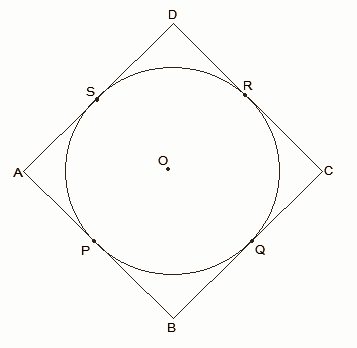ABCD is a parallelogram,

∴ AB = CD … (i)

∴ BC = AD … (ii)

From the figure,

DR = DS (Tangents to the circle at D)

CR = CQ (Tangents to the circle at C)

BP = BQ (Tangents to the circle at B)

AP = AS (Tangents to the circle at A)

DR + CR + BP + AP = DS + CQ + BQ + AS

⇒ (DR + CR) + (BP + AP) = (DS + AS) + (CQ + BQ)

⇒ CD + AB = AD + BC … (iii)

Putting the value of (i) and (ii) in equation (iii) we get,

2AB = 2BC

⇒ AB = BC … (iv)

By Comparing equations (i), (ii), and (iv) we get,

AB = BC = CD = DA

∴ ABCD is a rhombus.

Question 17: A chord of a circle of radius 10cm subtends a right angle at the centre. Find the area of the corresponding

[i] Minor segment

[ii] Major segment

Solution:

Angle = 90o

Area of sector A = 90o / 360𝛑r2

A = 3.14 x 10 x [10 / 4]

A = 25 x 3.14

A = 78.5sq.cm

Let the angle subtended and radius form an arc AOB, then

Area of AOB = r x r sin90o / 2

AOB = 10 x 10 x [1 / 2]

Area of AOB = 50sq.cm

Area of minor segment = 78.5 – 50 = 28.5 sq.cm

Then area of circle = 𝛑r2

= 3.14 x 10 x 10

= 314 sq.cm

Area of major segment = area of circle – area of minor segment

= 314 – 28.5

= 285.5 sq.cm

Question 18: A heap of rice is in the form of a cone of diameter 12m and height 8m. Find its volume. How much canvas cloth is required to cover the heap?

Solution:

Given, diameter = 12 m

Height = 8 m

The volume of rice in the heap = (1 / 3) πr²h

= (1 / 3) * (22 / 7) * (6)² * 8

= 301.71 m³

Now, l = √r² + h²

= √6² + 8²

= 10 m

∴ Canvas cloth required to cover the heap = πrl

= (22 / 7) * 6 * 10

= 188.57 cm²

GROUP – B

Question 19: If cosec θ + cot θ = k, then show that cos θ = [k2 – 1] / [k2 + 1].

Solution:

cosec θ + cot θ = k

[1 / sin θ] + [cos θ / sin θ] = k

[1 + cos θ] / sin θ = k

1 + cos θ = k sin θ

On squaring both sides,

(1 + cosθ)2 = k2 sin2θ

(1 + cosθ) (1 + cosθ) = k2 (1 – cos2θ) = k2 (1 + cosθ) (1 – cosθ)

1 + cosθ = k2 (1 – cosθ)

1 + cosθ = k2 – k2 cosθ

1 + (1 + k2) cosθ = k2

(1 + k2) cosθ = k2 – 1

cos θ = [k2 – 1] / [k2 + 1]

Question 20: Two men on either side of a temple of 30m height observe its top at the angles of elevation 30o and 60o respectively. Find the distance between the two men.

Solution:

Height of the temple AB = 30m

In right-angle triangle ABC

BC / AB = cot 30˚= √3

= BC / 30

= √3

= BC

= 30√3 ….(i)

Also in right-angled triangle ABD,

BD / AB = cot 60˚

= BD / 30

= 1/√3

= BD

= 30 / [√3 × √3 / √3]

= 10√3 ……(ii)

Thus, the distance between the two men = CD

= BC + BD

= 30√3 + 10√3

= 40√3

The distance between the two men will be 40√3 m.

Question 21: One card is drawn from a well-shuffled pack of 52 cards. Find the probability of getting

[i] a king of red colour

[ii] a face card

[iii] the jack of hearts

[iv] a red face card

[vi] the queen of the diamond

Solution:

Total Cards 52

i) king of Red colour – 2 King – (HEART & DIAMOND)

Probability of king of red colur = 2 / 52 = 1 / 26

ii) Face card = 4 * 3 = 12

Probability of Face Card = 12 / 52 = 3 / 13

iii) Red face Card = 2 * 3 = 6

Probability of Red Face Card = 6 / 52 = 3 / 26

iv) the jack of hearts = 1

Probability of the jack of hearts = 1 / 52

Probability of a spade = 13 / 52 = 1 / 4

vi) the queen of diamonds = 1

Probability of the queen of diamonds = 1 / 52

Question 22: The distribution below gives the weights of 30 students in a class. Find the median weight of the students.

 Weight (in kgs) 40 – 45 45 – 50 50 – 55 55 – 60 60 – 65 65 – 70 70 – 75 Number of students 2 3 8 6 6 3 2

Solution:

 Weight (in kgs) 40 – 45 45 – 50 50 – 55 55 – 60 60 – 65 65 – 70 70 – 75 Number of students 2 3 8 6 6 3 2 Cumulative frequency 2 5 13 19 25 28 30

Here, n = 30

n / 2 = 15

Since the cumulative frequency just greater than 15 is 19 and the corresponding class is 55 – 60. Therefore 55 – 60 is the median class.

Here, l = 55 , f = 6, c.f = 13, h = 5

MEDIAN = l + [(n / 2 – cf ) / f ] ×h

= 55 + [(15 – 13) / 6] × 5

= 55 +(2× 5) / 6

= 55 + 10 / 6

= 55 + 5 / 3

= 55 + 1.667

= 56.667 ≈ 56.67

Hence, the median weight of students is 56.67 kg.

SECTION – IV

(1 * 5 = 5)

Answer ANY ONE of the following questions.

Question 23: Construct a triangle of sides 4cm, 5cm, and 6cm, then construct a triangle similar to it, whose sides are (2 / 3) of the corresponding sides of the first triangle.

Solution:

Steps of Construction:

Step I: AB = 6 cm is drawn.

Step II: With A as a centre and radius equal to 4cm, an arc is drawn.

Step III: Again, with B as a centre and radius equal to 5 cm an arc is drawn on the same side of AB intersecting the previous arc at C.

Step IV: AC and BC are joined to form ΔABC.

Step V: A ray AX is drawn making an acute angle with AB below it.

Step VI: 5 equal points (sum of the ratio = 2 + 3 = 5) is marked on AX as A1 A2 …. A5

Step VII: A5B is joined. A2B’ is drawn parallel to A5B and B’C’ is drawn parallel to BC.

ΔAB’C’ is the required triangle

Justification:

∠A (Common)

∠C = ∠C’ and ∠B = ∠ B’ (corresponding angles)

Thus, ΔAB’C’ ~ ΔABC by AAA similarity condition

From the figure,

AB’ / AB = AA2 / AA5 = 2 / 3

AB’ = 2 / 3 AB

AC’ = 2 / 3 AC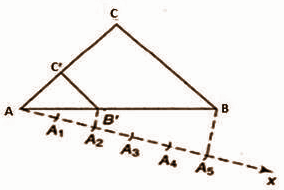Question 24: A tree breaks due to a storm and the broken part bends so that the top of the tree touches the ground by making 30o angle with the ground. The distance between the foot of the tree and the top of the tree on the ground is 6m. Find the height of the tree before falling down.

Solution: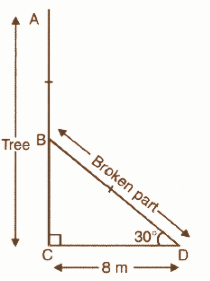Let the tree be AC and is broken at B. The broken part touches at point D on the ground. In right ΔBCD,

cos 30o = CD / BD

√3 / 2 = 8 / BD

BD = 16 / √3

tan 30o = BC / CD

1 / √3 = BC / 8

BC = 8 / √3

Height of tree = BC + BD

= 8 / √3 + 16 / √3

= 8√3m

The height of the tree is 8√3m.

PART – B

Choose the correct answer. (10 * 0.5 = 5)

Question 1: In figure DE || BC. Find the value of x.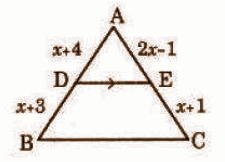(A) √5 (B) √6 (C) √3 (D) √7

Question 2: Volume of the cone is

(A) 𝛑rh (B) 𝛑rl (C) 𝛑r(r + l) (D) (1 / 3)𝛑r2h

Question 3: If the arithmetic mean of 8, 6, 4, x, 3, 6, 0, is 4; then the value of x is

(A) 7 (B) 6 (C) 1 (D) 4

Question 4: Probability of getting a prime or a composite number is

(A) Mutually exclusive (B) Likely (C) 0 (D) None

Question 5: Length of class 11 – 20 is

(A) 9 (B) 10 (C) 11 (D) 20

Question 6: The ratio of volumes of two spheres is 8:27, then the ratio of surface areas are

(A) 2:3 (B) 4:27 (C) 8:9 (D) 4:9

Question 7: In triangle ABC, ∠B = 90o, sin C = 3 / 5, then cos A =

(A) 3 / 5 (B) 4 / 5 (C) 5 / 4 (D) 5 / 3

Question 8: If a coin is tossed, then the probability that a head turns up is

(A) 1 / 2 (B) 1 / 4 (C) 1 / 3 (D) 1 / 6

Question 9: If x = sinθ, y = cosθ, then which of the following is true?

(A) x2 + y2 = 1 (B) x2 – y2 = 1 (C) x / y = 1 (D) xy = 1

Question 10: In the figure, if ∠APB = 60o and OP = 10cm, then PA = ___ cm.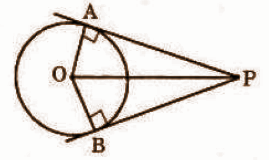(A) 5 (B) 5√2 (C) 5√3 (D) 20

Fill in the blanks with suitable answers. (10 * 0.5 = 5)

Question 11: Sum of central angles in a circle is _____ (36oo).

Question 12: Football is an example of _____ (sphere)

Question 13: Arithmetic mean of a – 2, a, a + 2 is ____ (a)

Question 14: P(E) + P(not E) = _____ (1)

Question 15: From the figure, the probability of getting a blue colour ball is ______ (0.6)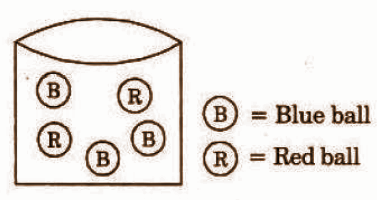Question 16: In triangle ABC, AC2 = AB2 + BC2, then the right angle is at ____ (B)

Question 17: Base area of a circular cylinder is 154cm2. Then its radius is ___ (7 cm)

Question 18: Curved surface area of a hemisphere with radius ‘r’ is _____ (2πr2)

Question 19: Arithmetic mean of 1, 2, x, 3 is 0, then x = ___ (-6)

Question 20: sin (60o + 30o) = ______ (1)

Question [i] Find the correct answer for the given question under group – A selecting from group – B. (10 * 0.5 = 5)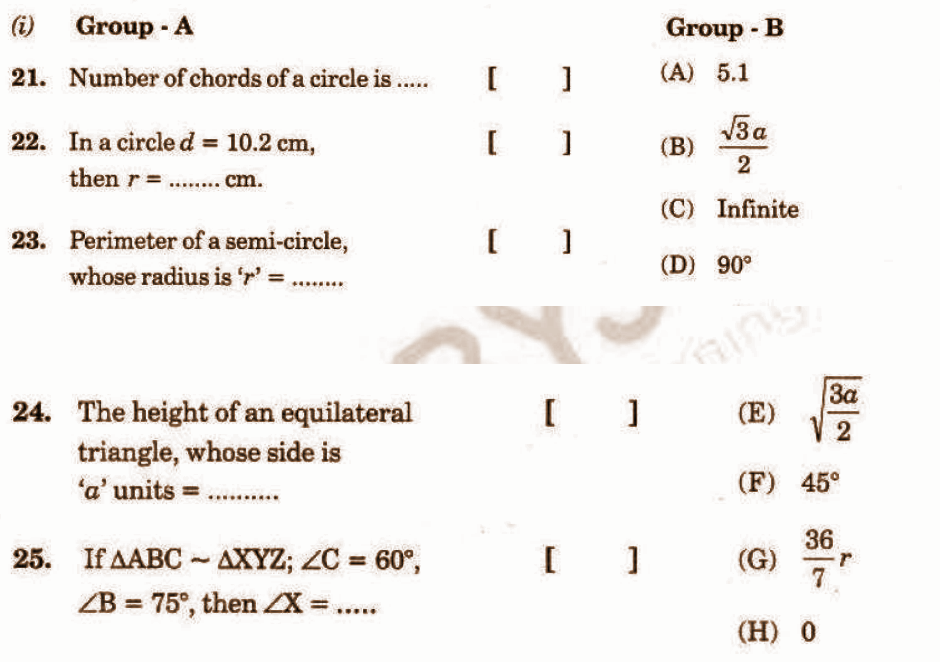21 – (C)

22 – (A)

23 – (G)

24 – (B)

25 – (F)

[ii]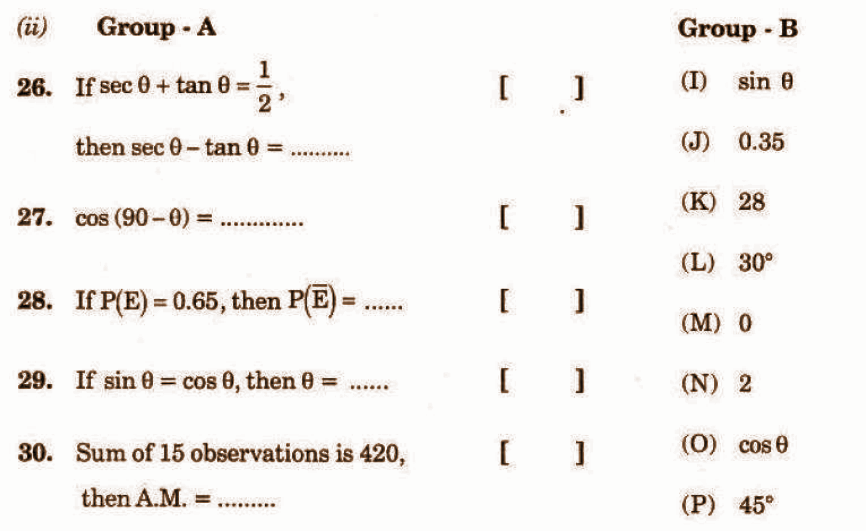26 – (N)

27 – (I)

28 -(J)

29 – (P)

30 – (K)